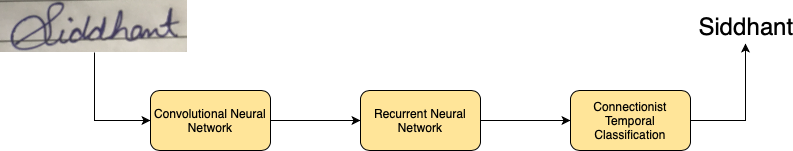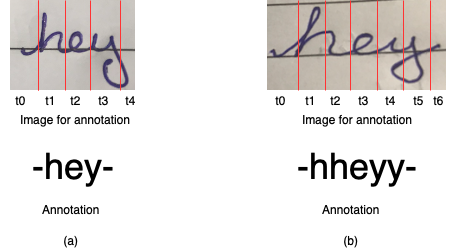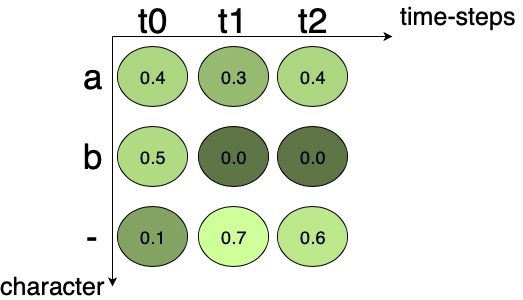Connectionist Temporal Classification (CTC) is a type of Neural Network output helpful in tackling sequence problems like handwriting and speech recognition where the timing varies. Using CTC ensures that one does not need an aligned dataset, which makes the training process more straightforward.Fig.1 Flow of Handwriting Recognition using CNN and RNN

## What does CTC do?

In the case of creating an OCR (Optical Character Reader), CRNN (Convolutional Recurrent Neural Networks) are a preferred choice. They output a character-score for each time-step, which is represented by a matrix. We now need to use this matrix for:

• Training the Neural Network, i.e., calculating the loss
• Decoding the output of the Neural Network

CTC operation helps in achieving both tasks.

## Problems solved by CTC

Imagine creating a dataset full of images of text and specifying each time-step of the image’s corresponding character, as shown in Fig2(a) There are a couple of issues with this approach:

• Annotating a dataset at the character level is a tedious task
• What if the character takes up more than one time-step? (as shown in Fig2(b)) It will result in duplication of the characters.Fig2 (a) Shows the annotation for a case when each character takes up one time-step (b) Shows the annotation for a case when a few characters take up more than one time-step

Here, CTC comes to the rescue:

• CTC is formulated in such a way, that it only requires the text that occurs in the image. We can ignore both the width and position of the characters in an image.
• There is no need for post-processing the output of the CTC operation! Using decoding techniques, we can directly get the result of the network.

## CTC Working

CTC works on the following three major concepts:

• Encoding the text
• Loss calculation
• Decoding

# Encoding the text

The issue with methods not using CTC is, what to do when the character takes more than one time-step in the image? Non-CTC methods would fail here and give duplicate characters.

To solve this issue, CTC merges all the repeating characters into a single character. For example, if the word in the image is ‘hey’ where ‘h’ takes three time-steps, ‘e’ and ‘y’ take one time-step each. Then the output from the network using CTC will be ‘hhhey’, which as per our encoding scheme, gets collapsed to ‘hey’.

Now this question arises: What about the words where there are repeating characters? For handling those cases, CTC introduces a pseudo-character called blank denoted as “-“ in the following examples. While encoding the text, if a character repeats, then a blank is placed between the characters in the output text. Let’s consider the word ‘meet’, possible encodings for it will be, ‘mm-ee-ee-t’, ‘mmm-e-e-ttt’, wrong encoding will be ‘mm-eee-tt’, as it’ll result in ‘met’ when decoded. The CRNN is trained to output the encoded text.

# Loss calculation

To train the CRNN, we need to calculate loss given the image and its label. We are getting a matrix of the score for each character at every time-step from the CRNN. Fig3 shows an example of an output matrix from the CRNN. There are three time-steps and three characters (including one blank). At each time-step, the character score sums up to 1.

For calculating the loss, all the possible alignments’ scores of the ground truth are summed up. In this manner, it is not significant where the character occurs in the image.Fig.3 Output matrix from the Neural Network. It shows the character probability at each time-step.

Corresponding character scores are multiplied together to get the score for one path. In Fig.3 the score for the path “a–” is 0.4x0.7x0.6 = 0.168, and for the path “aaa” is 0.4x0.3x0.4 = 0.048. For getting the score corresponding to given ground truth, scores of all the paths to the corresponding text are summed up.

For example, if the ground truth is “a”, all the possible paths for “a” in Fig.3 are “aaa”, “a–”, “a-“, “aa-“, “-aa”, “–a”. Summing up the score of the individual path we get, 0.048 + 0.168 + 0.018 + 0.072 + 0.012 + 0.028 = 0.346. 0.346 is the probability of the ground truth occurring, it is not the loss. As we know, the loss is the negative logarithm of probability, it can be calculated easily. This loss can be back-propagated and the network can be trained.

# Decoding

Once CRNN gets trained, we want it to give us output on unseen text images. Putting it differently, we want the most likely text given an output matrix of the CRNN. One method can be to examine every potential text output, but it won’t be very practical to do from a computation point of view. The best path algorithm is used to overcome this issue.

It consists of the following two steps:

• Calculates the best path by considering the character with max probability at every time-step.
• This step involves removing blanks and duplicate characters, which results in the actual text.

For example, let us consider the matrix in Fig.3. If we consider the first time-step t0, then the character with maximum probability is ‘b’. For t1 and t2 character with maximum probability is ‘-‘ and ‘-‘ respectively. So, the output text according to the best path algorithm for matrix in Fig.3 after decoding is ‘b’.

## Conclusion

Initially, we looked at the problems faced with a naive Neural Network for the handwriting recognition task. Then, we looked into how CTC can solve those issues. Then, we saw how CTC functions by encoding the text, method of calculating the loss, and decoding the output from a Neural Network trained using CTC.

This article covers the most basic version of the decoding process. There are other methods using which we can decode the output from the CTC-trained network. We will look into those methods in future article.